# Odlyzko bounds

Effective lower bounds for, the minimal value of the discriminantof algebraic number fieldshaving signature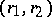(i.e. havingreal and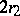non-real conjugates), obtained in 1976 by A.M. Odlyzko. See also Algebraic number; Number field.

The first such bound was proved in 1891 by H. Minkowski [a4], who showed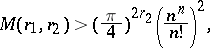(a1)

with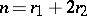. He obtained it using methods from the geometry of numbers; the same method was used later by several authors to improve (a1) (see [a5] for the strongest result obtained in this way).

In 1974, H.M. Stark ([a11], [a12]) observed that Hadamard factorization of the Dedekind zeta-functionleads to a formula expressing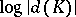by the zeros ofand the value of its logarithmic derivative at a complex number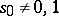with. He used this formula with a proper choice ofto deduce lower bounds for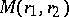which were essentially stronger than Minkowski's bound, but did not reach the bounds obtained by geometrical methods.

In 1976, Odlyzko [a7] (cf. [a9]) modified Stark's formula and obtained the following important improvement of (a1):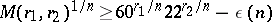(a2)

with.

In particular, one has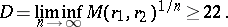If the extended Riemann hypothesis is assumed (cf. also Riemann hypotheses; Zeta-function), then the constants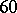andin (a2) can be replaced byand, respectively. For small degrees the bound (a2) can be improved (see [a2], [a10]) and several exact values ofare known.

On the other hand, it has been shown in [a13], as a consequence of their solution of the class field tower problem (cf. also Tower of fields; Class field theory), thatis finite. The best explicit upper bound for it,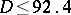, is due to J. Martinet [a1], who obtained this as a corollary of his constructions of infinite-class towers of suitable fields.

For surveys of this topic, see [a9], [a3] and [a8].